# Exponential law (in topology)

The idea for a topology on spaces of functions goes back to the metric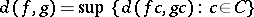on functions from a compact spaceto a metric space. It was found desirable to extend this to the case whenis only locally compact (cf. also Locally compact space).

To this end, R.H. Fox introduced the compact-open topology on the set of continuous functions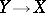, whereandare topological spaces (cf. also Compact-open topology; Topological space). This has a sub-base of sets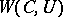forcompact inandopen in, whereis the set of continuous functionssuch that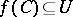. Fox also began the investigation of the relation of this to the "exponential law" .

The exponential law for sets uses the setof functions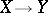and states that for any sets,,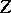there is a natural bijection, given by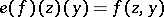,,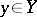. This law is an expression of the standard idea that a function of two variables can be thought of as a variable function of one variable.

Fox sought a similar result when,,are topological spaces and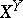is replaced by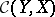, the set of continuous functions. This required finding an appropriate topology on. Unfortunately, it was found that this worked well only forlocally compact, in the sense of having a neighbourhood base of compact sets, and that the appropriate topology was the compact-open topology. A careful analysis of topologies onin relation to the exponential law was given by R. Arens and J. Dugundji.

The restriction to locally compact spaces for the validity of the exponential law was awkward for topology. It was suggested by E. Spanier in [a13] that the situation could be remedied by using "quasi-topological spaces" , which specify fora set of mappingsfor all compact Hausdorff spaces, satisfying appropriate axioms (cf. also Hausdorff space). This suggestion was subsequently felt to be vitiated by the fact that a two-point set had a class of quasi-topological structures (see the discussion and references in [a12]).

R. Brown in [a8] found that the exponential law was satisfied in the category of Hausdorff-spaces (cf. Space of mappings, topological) and continuous mappings. In [a2] it was suggested that this category "may be adequate and convenient for all purposes of topology" . The exposition in [a3] suggested the equivalent category of Hausdorff spaces and mappings continuous on compact subsets. It also explained the failure of the exponential law for all spaces, by giving a law of the formfor a new product topology.

The theme of "convenient categories" was also taken up in the expository paper [a14], again using Hausdorff-spaces, but called "compactly-generated spaces" .

It was known about that time (1967) that the Hausdorff condition could be removed by taking compactly generated to mean "having the final topology with respect to all mappings of compact Hausdorff spaces into the space" . In [a7] this is generalized to the case of certain classesof compact Hausdorff spaces, considering the set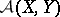of-continuous mappings between spaces, and giving this set a topology with a sub-base of sets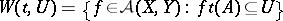for all open sets inand all "test" mappings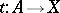for.

An important extension of results on the exponential law involves spaces of partial maps on closed subsets. A useful trick here is the representability of such partial mappings, an idea which comes from topos theory: The setof partial mappings with closed domain is bijective with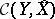where, where, andis closed in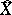if and only ifis closed inor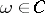— thus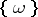is open but not closed in.

Using this device one can obtain an exponential law in the slice categoryof compactly generated spaces over the compactly generated spaceprovidedis a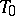-space (cf. Separation axiom). If,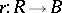are spaces over, then the space of functionshas as fibre over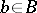the space of mappings. The topology is the join (in the given convenient category) of the topology on partial mappings with closed domain and that which makescontinuous. A consequence of the fibred exponential law is that a mapping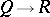overcorresponds exactly to a section of the mapping. This law has been extended to more general situations in [a6]. These laws are very useful tools in algebraic topology.

A dual device yields a topology on spaces of mappings with open domain [a1], but this has not yet (2000) been much exploited. This is surprising, since the solutions of many standard problems, such as differential equations, are often partial functions with variable open domain.

In [a11] the category of sequential spaces is embedded into a topos.

Approaches based on other kinds of set-open topologies and on graph topologies, with the aim of such applications, can be found in, for example, [a5], [a9], which point to a substantial literature in this area. However, [a15] uses categorical concepts and constructions to give a fairly comprehensive theory of differentiation in fairly general linear spaces of arbitrary dimension.

How to Cite This Entry:
Exponential law (in topology). Encyclopedia of Mathematics. URL: http://encyclopediaofmath.org/index.php?title=Exponential_law_(in_topology)&oldid=41971
This article was adapted from an original article by R. Brown (originator), which appeared in Encyclopedia of Mathematics - ISBN 1402006098. See original article